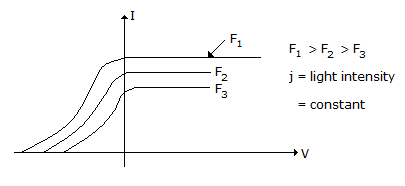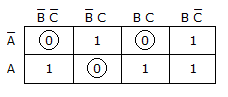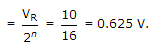# Electronics and Communication Engineering - Exam Questions Papers

21.

Consider the following

1. Maximum energy of electrons liberated photoelectrically is independent of light intensity
2. Maximum energy of electrons liberated photoelectrically varies nonlinearly with frequency of incident light.

 A. (1) is true and (2) is false B. (1) and (2) both are true C. (1) is false and (2) is true D. (1) and (2) both are false

Explanation:

Statement (ii) is false because maximum energy of photons varies linearly with frequency of incident light. It can be shown as follows :F1 > F2 > F3

j = light intensity = constant

Photocurrent Vs Anode voltage with frequency and incident light as a parameter.

The light intensity is constant.

22.

When phase-lag compensation is used in a system, then gain cross over frequency, bandwidth and undamped frequency are respectively

 A. Increased, Increased, Increased B. Increased, Increased, Decreased C. Increased, Decreased, Decreased D. Decreased, Decreased, Decreased

Explanation:

Phase lag is delay between a correcting signal of control system and response to it.

23.

The equivalent form of the logical expression ABC + A BC + ABC + ABC + AB C is

 A. C + (A ⊕ B) B. (A + B + C)(A + B + C) C. (A + B + C)(A + B + C)(A + B + C) D. (A + B + C)(A + B + C)(A + B + C)

Explanation:

This can be solve by using Boolean identity but using k map it will be more easy(A + B + C) (A + B + C) (A + B + C).

24.

The gradient of any scalar field always yields __________ .

 A. a solenoidal field B. a conservative field C. an irrotational field D. none of these

Explanation:

Dot product is conservative. Gradient is nothing but dot product.

25.

In a flash type ADC, employing 15 comparators resolution with 10 volts reference; is expected to be :

 A. 0.625 V B. 0.656 V C. 0.525 V D. insufficient data

Explanation:

Since number of comparators = 2n - 1 = 15;

Then number of bits are '4'

Resolution